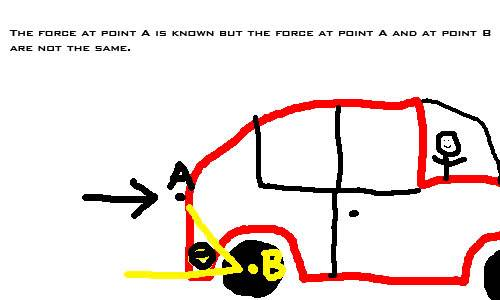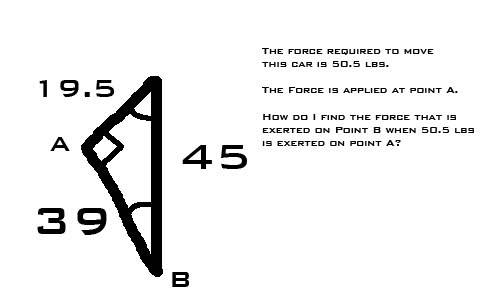# Trig - Can't rememeber Equation

Tonik
I'm unsure if this should be in homework help or not... but...

In Trig (over a year ago) I remember being taught a equation to find the force being applied (in this case) to the tires of a car when pushing it with "x" force at "y" height.

Lo and behold, I need this equation for a project I'm doing but I cant find it online or in my notes. Can anyone shine some light on this for me?

## Answers and Replies

Gregg
The question isn't clear

Tonik
Okay, sorry. I drew a [very poor] picture to help illustrate what I'm trying to get at.The force at point A will be known but it will not equal the force at point B. There will be some angle (theta) that I will approximate. I know that there is a relation between the two points along with the angle between the two but I can't figure out what the equation was.

Gregg
Ok you mean about resolving forces? the force is going to be A multiplied by the perpendicular distance.

But actually in that diagram the is no moment and I can't exactly see what you mean by the force at B?

Last edited:
Tonik
Okay I just did a little experiment and found dimensions and angles of this car I'm pushing.Basically this is the triangle that I drew here is what I'm pushing, only... in the form of a car. (point A and point B are the same points on both pictures.)

I'm trying to find the equation that show how much force will be applied to point B when I apply a force to Point A.

Ok you mean about resolving forces? the force is going to be A multiplied by the perpendicular distance.

But actually in that diagram the is no moment and I can't exactly see what you mean by the force at B?

I was under the impression that, since the wheel is moving instead of the actual car, the force is actually exerted to the wheel (point B) from the car (point A).

Also, I'm unsure what the perpendicular distance to a point of a triangle would be.

EDIT: I could just be going crazy and they be the same force... It is very possible.

Last edited:
Homework Helper
If you ignore all resistive forces, yes you are going crazy and both forces are the same :tongue:
Look at it in a slightly different way. Would it make any difference if you were pushing the car from the point A or parallel to the tyre at the point (lets say, C)? If you were pushing from the point C, isn't the point B now parallel and there is no trigonometry involved?

You're probably thinking trigo is needed because there are such things as having a force at an angle. For example, you could be pushing straight down on the top curved part of the car which would mean the force isn't parallel with the direction of movement. This means you are pushing as efficiently.

Tonik
yes you are going crazy

I knew it!

Thanks for the help everyone... I guess I'm just over thinking things for a relatively easy project.

Homework Helper
... easy project.

If you want to consider the pushing force, you better consider friction as well, else this easy project will be a very inaccurate project.

Susanne217
I'm unsure if this should be in homework help or not... but...

In Trig (over a year ago) I remember being taught a equation to find the force being applied (in this case) to the tires of a car when pushing it with "x" force at "y" height.

Lo and behold, I need this equation for a project I'm doing but I cant find it online or in my notes. Can anyone shine some light on this for me?

$$F = \Delta x \cdot cos(\theta)$$ Where can be written as $$F = N \cdot \mu_k$$